## research papersJOURNAL OFSYNCHROTRONRADIATION
ISSN: 1600-5775
Volume 26| Part 3| May 2019| Pages 793-800

## Depth-resolved compositional analysis of W/B4C multilayers using resonant soft X-ray reflectivity

aSynchrotrons Utilization Section, Raja Ramanna Centre for Advanced Technology, Indore 452013, India, bTechnical Physics Division, Bhabha Atomic Research Center, Trombay, Mumbai 400094, India, cUGC-DAE Consortium for Scientific Research, University Campus, Khandwa Road, Indore 452 001, India, and dHomi Bhabha National Institute, Anushakti Nagar, Mumbai 400094, India
*Correspondence e-mail: pnrao@rrcat.gov.in

(Received 26 October 2018; accepted 13 February 2019; online 5 April 2019)

W/B4C multilayers (MLs) consisting of ten layer pairs with varying boron carbide layer thicknesses have been investigated. The ML structures were characterized using grazing-incidence hard X-ray reflectivity (GIXR), resonant soft X-ray reflectivity (RSXR), hard X-ray photoelectron spectroscopy (HAXPES) and X-ray absorption near-edge spectroscopy (XANES). Depth-resolved spectroscopic information on the boron carbide layer in W/B4C MLs was extracted with sub-nanometre resolution using reflectivity performed in the vicinity of the B K-edge. Interestingly, these results show that the composition of boron carbide films is strongly dependent on layer thicknesses. HAXPES measurements suggest that most of the boron is in the chemical state of B4C in the multilayer structures. XANES measurements suggest an increase in boron content and C—B—C bonding with increase in boron carbide layer thickness.

### 1. Introduction

X-ray multilayers (MLs) consist of alternating layers of high- and low-electron-density elements (Spiller, 1994). ML elements are selected based on criteria, i.e. high optical contrast between the materials and low absorption. The most commonly used low-electron-density elements for application in the soft X-ray extreme-ultraviolet (SXR-EUV) regime are B, C, Mg, Al, Si, Sc and V. The high-electron-density elements like Cr, Ni, Mo, Ru, Rh, Pd, La and W are used as reflectors in combination with low-electron-density elements depending on the application wavelength. Among other MLs, Mo/Si is the most studied combination, because it finds potential application in EUV lithography for the operating wavelength of λ = 13.5 nm (Bajt et al., 2002). To further reduce the feature sizes, researchers are working on next-generation EUV lithography using radiation of shorter wavelength. The wavelength range around λ = 6.7 nm was preferred for next-generation EUV lithography (Makhotkin et al., 2012a). In this region B-based multilayers give the highest reflectivity because this region is within the anomalous dispersion of the optical constants of boron (Medvedev et al., 2013; Chkhalo et al., 2013; Tsarfati et al., 2009; Kuznetsov et al., 2015; Platonov et al., 2011). Boron carbide (B4C) is a promising spacer element for MLs that can be used for next-generation EUV lithography. However, the reported reflectivity of B4C-based MLs in the vicinity of the B K-edge is lower than the theoretical achievable values (Chkhalo et al., 2013). The maximum achievable reflectance from a ML combination depends on the structure and composition of the deposited layers (Makhotkin et al., 2012b). In particular, any compositional changes in the boron carbide layer due to either changes in stoichiometry or to the presence of impurities may have a detrimental effect on the optical properties of X-ray ML mirrors and the electronic properties of boron carbide/Si diodes (Rao et al., 2016; Lee & Dowben, 1994). For many applications, the boron carbide layer thickness is in the sub-nanometre to few tens of nanometres range. For example, MLs near the B K-edge required a period thickness of 3.3 nm and individual layer thicknesses of about 1–2 nm. Researchers also found that the period thickness changes in Mo/B4C and W/B4C MLs on annealing depending on the B4C layer thickness (Nyabero et al., 2013; Rao et al., 2015). Mo/B4C and W/B4C systems with a B4C layer thickness of less than 2 nm exhibit compaction, while the systems with a B4C layer thickness greater than 2 nm expand. It is thus important to study and quantify the atomic distribution of nanometre-scale buried layers with sub-nanometre depth resolution. In general, compositional information is extracted using ion or electron beam techniques. Electron beam techniques provide atomic compositional information about surfaces (Russell, 1999). However, not many techniques are available to extract the atomic composition information of nanometre thin films and interlayers formed at their interfaces with sub-nanometre depth resolution (Filatova et al., 2012).

A compositional change in boron carbide layers in ML structures has been reported earlier (Rao et al., 2016). Our recent study on boron carbide thin films showed a carbon-rich/boron-deficient growth as the film thickness reduces (Rao et al., 2018). To further investigate the compositional changes in boron carbide and its influence on the optical properties of ML structures, we have grown W/B4C MLs with varying boron carbide layer thickness. Grazing-incidence hard X-ray reflectivity (GIXR) is used to study structural properties. Soft X-ray reflectivity (SXR) measurements in the vicinity of the B K-edge were performed to investigate and to quantify the atomic distribution of deposited layers. The chemical states and relative content of boron in MLs were studied using hard X-ray photoelectron spectroscopy (HAXPES) and X-ray absorption near-edge spectroscopy (XANES) measurements. In this work we have chosen W/B4C MLs because W and B4C form stable interfaces and no interface reaction was observed at room temperature. As a result, we can ignore the interface width caused by chemical reaction between W and B4C which reduces the complexity of analyzing the measured resonant soft X-ray reflectivity (RSXR) data.

### 2. Theoretical background

The interaction of X-ray radiation with matter can be described using the energy-dependent complex refractive index n = 1 − δ + iβ, where δ (dispersion coefficient) and β (absorption coefficient) are the optical constants (Spiller, 1994). The optical constant δ describes the phase-shifting and dispersion properties of matter and β describes the absorption properties. Conventional hard X-ray reflectivity is one of many X-ray scattering techniques used to probe the structure of thin films and multilayers along the direction perpendicular to the sample surface. The quantity Δδ2 + Δβ2 is a measure of the reflection sensitivity between the two material phases (Ade & Hitchcock, 2008). In the hard X-ray region, the absorption is very small and it utilizes the difference in electron density between the materials, i.e. it is only sensitive to the phase shift related to δ. The modulations in the reflectivity pattern due to electron density difference were analyzed to obtain structural parameters like thickness, root-mean-square (r.m.s.) interface and surface roughness. For many of the light elements like C, B, Be, Si, Al and Mg the electron density difference is very small and cannot be distinguished using conventional hard X-ray reflectivity. In the vicinity of the absorption edge both the dispersive and absorption properties of matter are important. For many light elements the absorption edge falls in the extreme ultraviolet/soft X-ray region. RSXR utilizes both the phase shift and absorption sensitivity of matter and can be selectively employed by tuning the energy of the incident radiation. Thus analysis of RSXR data provides quantitative chemical compositional information similar to absorption spectroscopy (Nayak & Lodha, 2011). The angular dependence inherent to reflectivity measurements gives rise to furthermore spatial selectivity. The optical constants of multi-element compounds can be written as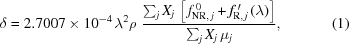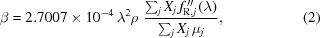where λ is the incident wavelength (nm), ρ is the density (g cm−3), Xj is the atomic fraction of j atoms and μj is the atomic weight of the j atoms (g mol−1), fNR0(q) is the non-resonant atomic scattering factor (ASF), and fR′(λ) and fR′′(λ) are the dispersion and absorption corrections to the ASF arising from the bounded electrons in an atom. We can enhance the optical contrast between two similar electron density elements by selectively employing the incident photon energy, and can distinguish between them using RSXR.

### 3. Experimental techniques

#### 3.1. Sample preparation

W/B4C MLs consisting of ten layer pairs of different period thicknesses were deposited on an ultrasonically cleaned Si (100) wafer using the ion beam sputtering technique. Four ML structures with B4C layer thicknesses dB4C ≃ 1.3, 1.9, 2.8 and 4.0 nm were deposited. The thickness of the W layers was kept at around 2.7 nm in all MLs. These MLs were named as ML-1 (dB4C = 1.3 nm), ML-2 (dB4C = 1.9 nm), ML-3 (dB4C = 2.8 nm) and ML-4 (dB4C = 4.0 nm). A commercially available four-inch sputtering target of 99.95% purity for W and 99.5% purity for B4C was used for the deposition. The base pressure of the system was ∼3 × 10−5 Pa. During the deposition, Ar gas flow was fixed at 3 standard cubic centimetres, which results in a vacuum drop to 6 × 10−2 Pa in the chamber. An Ar ion beam of current ∼25 mA and voltage 1000 V was used to sputter the target material. Target materials were pre-sputtered for about 30 min before the deposition of MLs.

#### 3.2. Reflectivity measurements

GIXR measurements were carried out using a Bruker Discover D8 diffractometer and Cu Kα radiation (λ = 0.154 nm). GIXR measurements were performed using a 100 µm slit for the incident beam and a 200 µm slit for the reflected beam. The angle-dependent soft X-ray reflectance measurements were performed in the wavelength regime 6.3–6.8 nm using the reflectivity beamline at the Indus-1 synchrotron facility (Nandedkar et al., 2002). This beamline has a toroidal grating monochromator and delivers photons in the range 4–100 nm with high flux (∼1011 photons s−1) and a moderate spectral resolving power (λ/Δλ) of 200–500. Various absorption-edge filters are provided in the beamline to suppress the higher-order contamination from the monochromator. A nonlinear least-squares curve-fitting technique based on the χ2 minimization method was applied for determination of the structural parameters and optical constants from the reflectivity curves (Parratt, 1954).

#### 3.3. HAXPES

HAXPES measurements were carried out on beamline BL-14 at the Indus-2 synchrotron radiation source (Jagannath et al., 2018), and performed using an excitation energy of 4404 eV. The photoelectron spectra were measured at an electron emission angle θ = 45°. An inelastic mean free path (IMFP; Λ) of the emitted photoelectrons with such an excitation energy can be expected to be 4.2–6.3 nm depending on the element and the core level (Tanuma et al., 2011). The maximum probing depth is defined as (3Λcosθ), which is approximately equal to two to three times the periods of the MLs. HAXPES measurements allow a larger depth to be probed than for X-ray photoelectron spectroscopy (Filatova et al., 2017, 2018). However, the 1s core levels of the elements B (3.0 × 10−26 m2), C (5.2 × 10−26 m2) and O (1.7 × 10−25 m2) have smaller photoionization cross sections at the excitation energy 4404 eV (Scofield, 1973).

#### 3.4. XANES

XANES measurements near the B K-edge were performed at the soft X-ray absorption beamline (BL-01) at the Indus-2 synchrotron radiation source (Phase et al., 2014). The XANES data were collected in total electron yield (TEY) mode by recording drain current from the sample. The energy resolution at BL-01 at the B K-edge energy is better than 300 meV.

### 4. Results and discussions

#### 4.1. GIXR

Reflectivity curves obtained from GIXR measurement at the Cu Kα wavelength (λ = 0.154 nm) along with theoretically calculated curves as a function of scattering vector qz (= 4πsinθ/λ, where θ is the grazing-incidence angle and λ is the wavelength of probing beam) are presented in Fig. 1. The reflectivity pattern contains small oscillations followed by maxima at certain scattering wavevectors. These small oscillations are the Kiessig fringes, which are caused by the interference between the waves reflected at the free surface and at the substrate surface, whereas the Bragg maxima are due to the in-phase addition of reflections from each interface. The observed Bragg peak reflectivity, width and the rate at which reflectivity falls depend upon the deposited layer thicknesses, densities and interface widths. To extract these structural parameters, we simulate the experimentally measured reflectivity data with a two-layer model consisting of W and B4C. The difference between the experimentally measured and the calculated reflectivity is minimized by varying the layer thicknesses (d), densities (ρ)/optical constants and interface width for the W-on-B4C (σ1) and the B4C-on-W (σ2) interfaces. The density of the B4C layer is estimated to be 2.2 g cm−3 and that of the W layer is estimated to be 15.7 g cm−3 in all four MLs. Since the mass densities of W and B4C are significantly different, the average mass density of the W embedded in B4C layer increases significantly and can be observed in the GIXR profile. In W/B4C MLs, researchers have found that there is an increase in the B4C density due to a discontinuity of the B4C layer and interdiffusion of W atoms into the B4C layer as the layer thicknesses reduces (Rai, 2013; Walton et al., 1998; Pradhan et al., 2016). In the present study, we did not observe any increase in density of the B4C layer with decrease in thickness, which suggest that the B4C layer is continuous and with no diffusion of W atoms into it. However, the densities of the deposited layers are lower than for the bulk values (2.52 g cm−3 for B4C and 19.3 g cm−3 for W). In general, sputter-deposited layers have densities lower than the bulk values due to presence of porosity and terminating the layer thickness before it reaches the close-packed structure (Chopra, 1969). The structural parameters obtained from the best-fitted models shown in Fig. 1are presented in Table 1. As seen from Table 1, the total interface width increases from 0.06d to 0.1d with a decrease in the period thickness. Measuring and simulating the reflectivity data at shorter wavelength provides the complete structural information. However, GIXR measurements are insensitive to the change in the B and C atomic percentage (at%) of the boron carbide layer because of the low electron density difference (∼2%) between these two elements. Hence reflectivity measurements in the vicinity of the B K-edge (6.6 nm) were performed to investigate the compositional changes in the boron carbide layer of W/B4C MLs.

 Table 1Structural parameters of W/B4C MLs obtained from the best-fitted models shown in Fig. 1
 ML period and layer thicknesses are estimated within an accuracy of 0.01 and 0.05 nm, respectively.
Sample name Period thickness, d (nm) dW (nm) dB4C (nm) Total interface width, σ = (σ12 + σ22)1/2 (nm)
ML-1 4.10 2.75 1.35 0.40
ML-2 4.60 2.70 1.90 0.39
ML-3 5.43 2.60 2.83 0.42
ML-4 6.75 2.70 4.05 0.40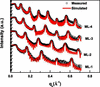Figure 1 Measured and simulated GIXR curves of all four W/B4C ML samples.

#### 4.2. RSXR

The sensitivity of RSXR to the compositional changes in the boron carbide layer in the vicinity of the B K-edge (6.6 nm) is shown in Fig. 2through simulations on the W/B4C ML structure. To calculate the optical constants of a multi-element layer we require the atomic scattering factors (ASFs) of each element (Rao et al., 2010, 2016, 2018). We used ASFs extracted from the optical constants of boron carbide thin films, measured by Soufil et al. (2008) for B and Henke et al. (1993) tabulated ASFs which work well away from the absorption edges for C. Any change in the composition of the boron carbide layers causes a significant change in the optical constants in the vicinity of the B K-edge. The change in optical constant causes the change in optical contrast between the W and boron carbide layers, which results in a significant change in the peak reflectance. As seen from Fig. 2, the peak reflectance decreases with a decrease in the B at% in the boron carbide layer and the variation in peak reflectance is less when crossing the B K-edge from lower to higher wavelength regime. In the vicinity of the B K-edge, C being a non-resonant atom has a flat response to the incident radiation, whereas B atoms, being resonant, have a strong response to the incident radiation. Any decrease in B content in the boron carbide layer reduces the optical contrast (≅ Δδ2 + Δβ2, where δ and β are called optical constants) between the W and boron carbide layer which results in a drop in reflectivity and a small change in peak reflectance when one moves across the absorption edge. So performing reflectivity measurements in the anomalous dispersion of the optical constants of boron gives an idea about the compositional changes in the boron carbide layer. The measured RSXR curves of W/B4C ML structures ML-1 and ML-2 in the vicinity of the B K-edge are presented in Fig. 3. From Fig. 3, we make the following observations: (i) there is no change in Kiessig fringe pattern and a very small variation in the peak reflectance in the case of ML-1, (ii) there is a significant change in the Kiessig fringe pattern in the case of ML-2 and also a considerable change in the peak reflectance when we move across the absorption edges, and (iii) there is a hump in the reflectivity pattern of ML-1 at lower qz (0.06 Å−1). A small increase in peak reflectance from 0.4% (at 6.30 nm) to 0.7% (at 6.72 nm) and no modulation in the Kiessig fringes when crossing the B K-edge from the lower to higher wavelength regime suggests that only a very small amount of B is present in sample ML-1. In the case of sample ML-2, the peak reflectance increases from 0.3% (at 6.30 nm) to 1.1% (at 6.72 nm), and modulation in the Kiessig fringes when crossing the B K-edge from the lower to higher wavelength regime suggests a significant increase in B at% compared with sample ML-1. The boron carbide layer thickness is 1.35 nm in sample ML-1 and 1.90 nm in sample ML-2. In order to observe compositional changes in such nanometre-thickness film we need techniques which can provide in-depth information with sub-nanometre resolution. The minimum resolvable feature in a reflectivity profile can be estimated by dmin =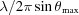= 2/qmax, where θmax and qmax are the maximal measured angle and scattering vector, respectively. For the reflectivity measurements presented in Fig. 3, qmax = 0.18, the minimum resolvable feature in the optical constant profile that can be constructed using a reflectivity profile is limited to ∼1.2 nm. However, features smaller than dmin are observed in the reflectivity curve and can be reconstructed in the optical constant profile. This fact is only explained by the interference nature of the reflection from an inhomogeneous media (Kozhevnikov, 2003). We can reconstruct the compositional profile of ML structures with sub-nanometre depth resolution by analyzing the RSXR data. Further, we observed a hump in the reflectivity curves of sample ML-1 at lower qz (0.06 Å−1) whereas such a feature is not visible in other ML samples. The reason for such an observed feature is not yet known clearly. The observed hump might be due to oxidation of the top layers in the ML structure. To explain this, we have assumed a structural model consisting of nine layer pairs and an oxidation layer on top while analyzing the measured RSXR data.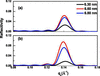Figure 2 Calculated RSXR curves of an ideal W (2.0 nm)/B4C (2.5 nm) ML structure consisting of ten layer pairs varying the boron carbide layer composition [(a) 60 at% B, 40 at% C and (b) 90 at% B, 10 at% C] at selected wavelengths in the vicinity of the B K-edge.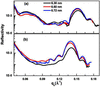Figure 3 Measured RSXR curves of W/B4C ML samples ML-1 (a) and ML-2 (b) at selected wavelengths in the vicinity of the B K-edge.

The measured RSXR curves of W/B4C ML structures ML-3 and ML-4 in the vicinity of the B K-edge are presented in Fig. 4. In the case of sample ML-3, the peak reflectance increases from 0.5% (at 6.30 nm) to 2.7% (at 6.72 nm) when crossing the B K-edge from lower to higher wavelength. In the case of sample ML-4, the peak reflectance increases from 1.2% (at 6.30 nm) to 7.2% (at 6.72 nm) when crossing the B K-edge from lower to higher wavelength regime. In both samples we have observed a significant variation in the peak reflectance and modulation in the Kiessig oscillations.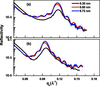Figure 4 Measured RSXR curves of W/B4C ML samples ML-3 (a) and ML-4 (b) at selected wavelengths in the vicinity of the B K-edge.

The measured and simulated RSXR curves of all four W/B4C ML samples at wavelength λ = 6.64 nm are presented in Fig. 5. The structural parameters, i.e. thickness and interface width, obtained from GIXR are used as input parameters while analyzing the RSXR data and the best fit is obtained by changing the optical indices of the layers. This approach reduces the number of free parameters in a fitting, which improves the reliability of the derived optical indices and hence the reconstructed composition profile of the layers. Soufli et al. (2008) measured optical constants for boron carbide thin films and Henke et al.'s (1993) tabulated optical constants for W are used as initial input parameters for our fitting. A thin capping layer at the surface of the ML structure, which is due to the ML sample being exposed to air, is also included in fitting the RSXR data. Fig. 6shows the profile of the optical index δ of all four samples as a function of depth obtained from the best-fitted model shown in Fig. 5. As can be seen from Fig. 6, the optical contrast between the W and B4C layer is enhanced with an increase in the thickness of the boron carbide layer. The optical contrast change is due to compositional changes in the boron carbide layer. This indicates that the RSXR measurements are sensitive to any compositional changes in the boron carbide layer on the sub-nanometre scale. At a wavelength of λ = 6.64 nm, B is a resonating atom, whereas C and O are non-resonating atoms. The polarization of the resonating atom is out of phase with the electric field, which results in the real part of the ASF and consequently δ becoming negative. The polarization of the non-resonating atoms is in phase with the electric field which results in the real part of the ASF and consequently δ becoming positive. The increase in the value of the optical constant suggests that there is a decrease in the B atomic concentration in the film, with a decrease in the film thickness. For example, the optical constant δ of the boron carbide layer changes from −0.00205 to 0.00465 when its composition changes from 85 at% B, 10 at% C and 5 at% O to 35 at% B, 60 at% C and 5 at% O. We assumed 5 at% O in calculating the optical constants as its presence is confirmed by resonant Rutherford backscattering spectrometry measurements performed on boron carbide thin films deposited using a similar system (Rao et al., 2018). From Fig. 6, it is clear that the optical constant δ (= 0.0140) of the W layer is the same in all ML samples and deviates from the tabulated value (0.0160) (Henke et al., 1993). The observed discrepancy is due to the density of the W films being lower than the bulk values as observed in GIXR. The optical constant δ of the boron carbide layer varies from −0.000456 to 0.00421 in the W/B4C ML samples. The compositions which give the best fit to the measured RSXR data of all four ML samples are presented in Table 2. As can be seen from Table 2, the B at% increases with increasing film thickness. However, there is a little uncertainty in estimating the relative atomic concentration of C and O, because the reflectivity measurements performed near the B K-edge are less sensitive to changes in the relative atomic concentration of these elements. The reduction of B concentration in the boron carbide layer causes a smooth variation in the optical constants, which limits the maximum achievable reflectance from the boron carbide based MLs near the B K-edge.

 Table 2Composition of the boron carbide layer in W/B4C MLs estimated from RSXR
Sample name Composition
ML-1 40 at% B, 55 at% C, 5 at% O
ML-2 55 at% B, 40 at% C, 5 at% O
ML-3 66 at% B, 29 at% C, 5 at% O
ML-4 74 at% B, 21 at% C, 5 at% O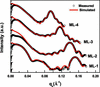Figure 5 Measured and simulated RSXR curves of W/B4C ML samples at 6.64 nm wavelength. The reflectivity curves have been shifted upwards for the sake of clarity.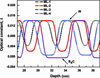Figure 6 Optical constant δ profile of W/B4C ML samples obtained from the best fit of RSXR data shown in Fig. 5.

The observed boron-deficient/carbon-rich growth is due to dissociation of the boron carbide target into individual B and C atoms in sputtering and recombination of these atoms on a given surface. Recombination on a surface depends on a large number of parameters, such as: the sticking coefficient, which depends on substrate temperature, roughness and chemistry; chemical bonds between the arriving atoms; reactive gases present in the chamber; velocity and angle of vapour atoms on a substrate; impurities present on the surface; etc. The sticking coefficient of B and C atoms are expected to be different on a given surface which causes the film composition to be different from the target composition (Chopra, 1969). Caretti et al. (1998) also observed that the number of carbon atoms incorporated is larger than the number of incident atoms in the boron carbide films formed by co-evaporation of B and C atoms. The higher sticking of C atoms is due to chemical bonding between B and C atoms.

#### 4.3. HAXPES

The survey HAXPES spectra of all four W/B4C ML samples are presented in Fig. 7. The spectra have been shifted vertically upward for better visibility. The HAXPES survey spectra depict contributions for W, O, C and B. The core-level C 1s photoemission spectra are not presented here because of hydrocarbon contamination present on the sample surface due to exposure to air dominating the photoemission spectra (Wu et al., 2018). Such a contaminated layer on top of the ML structure is also assumed while fitting the RSXR data. The core-level spectra of B 1s of all samples together with the spectrum deconvolution and Shirley background is shown in Fig. 8. As the B 1s core level has a small photoionization cross section, the data are taken for about 50 scans to improve the signal. The photoemission spectra are decomposed in order to obtain the different chemical states of B in the ML structures. The B 1s peak is fitted with two different chemical states at 188.5 eV and 193.0 eV. The first corresponds to B—C bonds while another is assigned to boron oxide. The contributions of boron oxide to the B 1s line are ∼30% and ∼16% for the ML samples ML-1 and ML-2, respectively. In the case of samples ML-3 and ML-4 we found the contribution of boron oxide to the B 1s line to be ∼10%. HAXPES results indicate that boron is in the chemical state of B4C and B2O3 while the contribution from boron oxide decreases with increasing boron carbide layer thickness in the ML structures.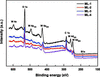Figure 7 Survey HAXPES spectra of all four W/B4C multilayer samples. The spectra have been vertically shifted for better visibility.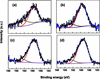Figure 8 B 1s core-level photoemission spectra of W/B4C ML samples ML-1 (a), ML-2 (b), ML-3 (c) and ML-4 (d) together with the deconvolution spectra and Shirley background.

#### 4.4. XANES

The B K absorption spectra of four W/B4C MLs are presented in Fig. 9. Pre- and post-edge normalization has been carried out using the Athena software package (Ravel & Newville, 2005). The prominent features in the B K absorption spectra are marked as a, b and c and appear at photon energies of ∼191.0, ∼192.2 and ∼193.8 eV, respectively. The peaks ab and c appear in all the samples with different relative intensities. The narrow edge peak b (∼192.0 eV) occurs due to the transition of a B 1s electron to the unoccupied B 2p π* state. The other narrow peak c (∼194.0 eV) is due to transition of a B 1s electron to the unoccupied B 2p π* state in B in the presence of oxygen (Jia et al., 1996; Zhang et al., 2000). Also, the peak a (∼191.0 eV) is observed which corresponds to bonding within the C—B—C chain. The measured absorption spectra are different from that of commercially obtained B4C powder (Jiménez et al., 1998) which indicates possible stochiometric changes during the deposition. The features in the high-energy regime (195–205 eV) are due to the transition of B 1s electrons to the unoccupied B σ* states. The presence of oxygen in the boron carbide layers is consistent with our earlier studies performed on boron carbide thin films (Rao et al., 2018). The features a and b become enhanced as the thickness of the boron carbide layer in the MLs increases, which suggests an increase in boron content and bonding with C atoms.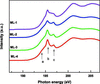Figure 9 XANES spectra near the B K-edge of all four W/B4C ML samples measured in TEY mode.

### 5. Conclusions

In conclusion, soft X-ray reflectivity measurements performed in the vicinity of the B K-edge were used to estimate the composition of the boron carbide layer in ML structures quantitatively. The optical constant profiles derived from the reflectivity spectra are sensitive to compositional changes on the sub-nanometre scale. RSXR measurements reveal significant changes in the composition of the boron carbide layer with thickness. The boron atomic percentage increases from 40 to 74 as the layer thickness increases from 1.4 nm to 4.0 nm. Such a compositional change in the boron carbide layer has adverse effects on the optical properties and limits the maximum achievable reflectance from boron carbide based multilayers. HAXPES measurements are performed to study the chemical states of B in different ML structures. HAXPES results indicate that boron is in the chemical states of B4C and B2O3 and the contribution from boron oxide to the B 1s photoemission peak decreases with increasing thickness of the boron carbide layer in the multilayer structures. XANES measurements suggested an increase in the B content with an increase in layer thickness. Unlike the conventional ion and electron beam spectroscopy techniques, the present approach has the advantage that it provides in-depth information in a non-destructive way and the angular dependence inherent to reflectivity brings further spatial selectivity with sub-nanometre resolution.

### Acknowledgements

The authors thank R. Dhawan for his help with the sample preparation. Thanks are due to M. H. Modi and R. K. Gupta for their help with the soft X-ray reflectivity measurements.

### ReferencesJOURNAL OFSYNCHROTRONRADIATION